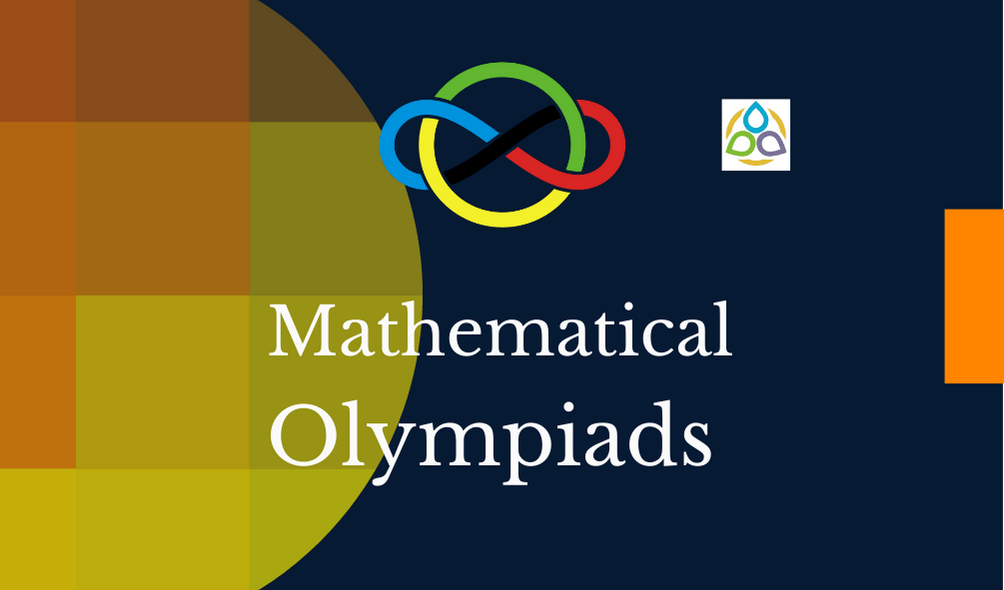# Miscellaneous Problems

Problem 31. Find an infinite set of positive integers such that the sum of any finite number of distinct elements of the set is not a square.

Problem 32. Which numbers have an odd number of divisors?

Problem 33. Let p be a prime number. List all the divisors of p2.

Problem 34.

Problem 35.

1.DEB JYOTI MITRA says:
1.Math1089 says: